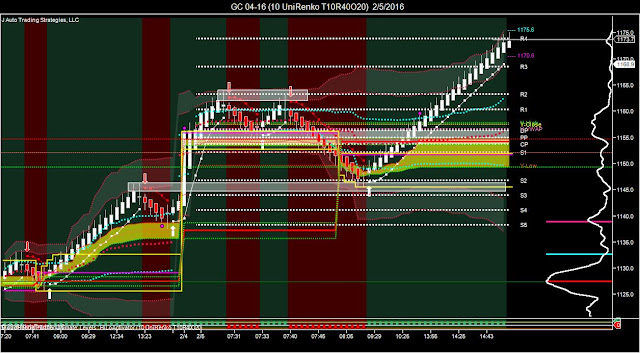## What's next for Gold?

Resistance:  1170, 1184, and 1193
POC at 1140
Support at 1122, 1115

With this strong momentum, I'm looking for 1193.Gann HiLo Solver
The Gann HiLo Indicator was introduced by Robert Krausz with FibonacciTrader in "The New Gann Swing Chartist," that was published in 1988's edition of TASC magazine (Stocks & Commodities V16:2, pp 57-66). The indicator uses a three day simple moving average calculated from the daily highs and the daily lows. JATS has customized these settings to get a reversal signal when the bar closes below the low average in an uptrend or above the high average in a downtrend.  The indicator value is calculated from the prior bar.  You can trade the signal inflections inside a larger trend direction for maximum value.  The indicator's stop line calculations can be taken from the following moving averages.

- a moving median
- a 2-pole Butterworth filter
- a 3-pole Butterworth filter
- a DEMA (double exponential MA)
- a DSMA (double simple MA)
- a DTMA (double triangular MA)
- a DWMA (double weighted MA)
- an Ehlers filter
- an EMA
- a 2-pole Gauss filter
- a 3-pole Gauss filter
- a 4-pole Gauss filter
- a HMA (Hull MA)
- a Holt EMA
- a Linear Regression indicator
- a SMA
- a 2-pole Supersmoother
- a 3-pole Supersmoother
- a TEMA (triple exponential MA)
- a TMA (triangular MA)
- a TSMA (triple simple MA)
- a TWMA (triple weighted MA)
- a VWMA(volume weighted MA)
- a WMA (weighted MA)
- a ZeroLagHATEMA
- a ZeroLagTEMA
- a ZLEMA